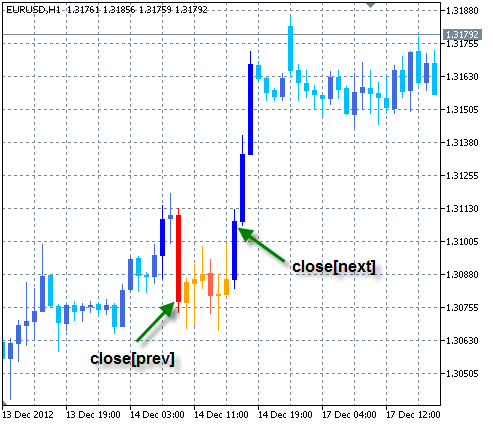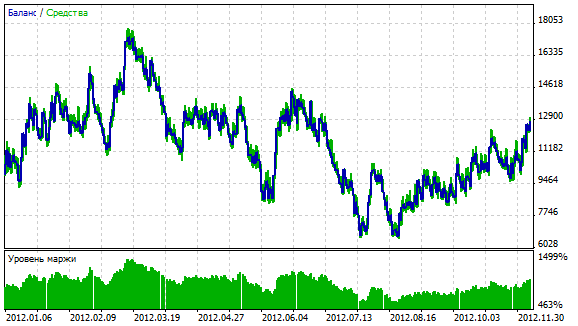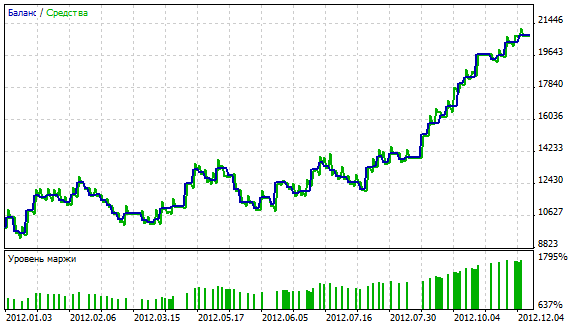Interesting script?
So post a link to it -
let others appraise it

You liked the script? Try it in the MetaTrader 5 terminal# Breakout Bars Trend EA - expert for MetaTrader 5

Views:
9015
Rating:
Published:
2013.01.14 11:59
Updated:
2016.11.22 07:32

For the Expert Advisor work you need the BreakoutBarsTrend_v2 custom indicator (download in the MQL5/Indicators folder, and the given Expert Advisor is in the MQL5/Experts folder).

The meaning is in the following:

• the position opens in the trend reversal. If the "Number of false signals" parameter is equal to zero, the position opens on each reversal. If this parameter is more than zero, the position will be opened only if the current reversal trend preceded the set number of false signals.
• position closes either when trend changes its direction, or stop loss/take profit.

The signal is considered to be false if the distance in pips from close price of the first bar of the trend to the close price of the next trend is negative. The example of the false trend to sell (close[prev] - close[next] < 0, for the false trend to buy close[next] - close[prev] < 0):Parameters:

• Reversal - reversal mode (in pips or percents)
• Delta - the minimum distance for trend change.
• Number of false signals - the positions will be opened only if the current signal has the set number of false signals. If the parameter is equal to zero, the position opens on each reversal.
• Besides the above parameters there are Stop-loss, Take-profit, Lot.

Note! If the reversal mode is in percentage, the parameters of Delta, Stop-loss and Take-profit also must be set in percentage. If the reversal is in pips, then Delta, Stop-loss and Take-profit also must be set in pips!

The testing results at EURUSD H1, 2012. Reversal in percentage, Delta = 0.3%, stop-loss and take profit are equal to 1%.

a) The entry on each reversal (Number of false signals is equal to 0)b) The same parameters, but the entry is after a series of false signals (Number of false signals is equal to 3)Translated from Russian by MetaQuotes Software Corp.
Original code: https://www.mql5.com/ru/code/1397The class for drawing the Momentum using the ring buffer

The class is designed for calculation the technical indicator Momentum (Momentum) using the algorithm of the ring buffer.TrendEnvelopes

The typical semaphore trend indicator.Exp_ColorXADX

The trading system based on change of the trend direction and force of the trend displayed by the ColorXADX indicator.Exp_MultiTrend_Signal_KVN